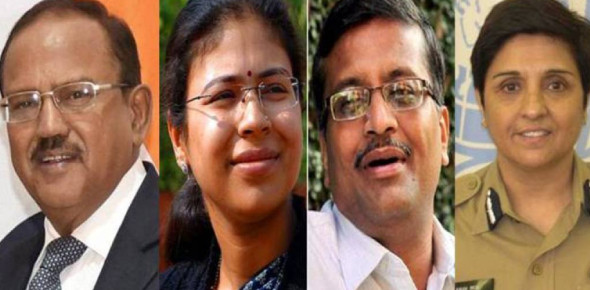# Indian Civil Services Online Test Quiz!

50 Questions | Attempts: 117
ShareSettings.

• 1.
The powers that contested for supremacy with rashtrakutas in north India were
• A.

Palas & Pratiharas

• B.

Pratiharas and Paramaras

• C.

Vengi chalukyas & Pallavas

• D.

Palas & Pallavas

• 2.
The Hoysala kingdom was finally absorbed into the kingdom of?
• A.

Bahmani

• B.

Pratiharas

• C.

Vijayanagar

• D.

Pallavas

• 3.
Chola king Kulottunga I earned fame by abolishing:
• A.

Sati

• B.

Toll tax

• C.

Slavery

• D.

Forced labour

• 4.
Pala kings were followers of?
• A.

Jainism

• B.

Buddhism

• C.

Hinduism

• D.

Islam

• 5.
'Jazia' was a tax on:
• A.

Non Muslims

• B.

Muslims

• C.

Hindus

• D.

Non Hindus

• 6.
Which of these was not a temple architecture style?
• A.

Nagara

• B.

Dravida

• C.

Vasara

• D.

Ghatika

• 7.
Who were samanthas?
• A.

King

• B.

Small chief

• C.

Servant

• D.

Rajaputs

• 8.
What was the main feature of the Cholas administration?
• A.

Military Organisation

• B.

• C.

• D.

Art and Architecture

• 9.
A group of villages during the Cholas period were called?
• A.

Tan Kurram

• B.

Kudavolai

• C.

Kottam

• D.

Variam

• 10.
Group of Kurrams during the Cholas period were called?
• A.

• B.

• C.

Vishaya

• D.

Udankuttam

• 11.
The Chola empire was divided into provinces called?
• A.

Vishayas

• B.

Aharas

• C.

Bhukties

• D.

Mandalams

• 12.
Village assemblies during the Cholas period were functioned through the committees called?
• A.

Variams

• B.

Kottams

• C.

Kurrams

• D.

• 13.
Who was the last ruler of the Chola dynasty?
• A.

• B.

• C.

• D.

• 14.
What would happen if human blood becomes acidic?
• A.

Oxygen carrying capacity of haemoglobin is decrease

• B.

Oxygen carrying capacity of haemoglobin is increase

• C.

Will be Unpredictable

• D.

Will remain same

• 15.
The absorption of ink by blotting paper involves:
• A.

Capillary action phenomenon

• B.

Diffusion of ink through the blotting

• C.

Viscosity of ink

• D.

Siphon action

• 16.
Which of the following parameters does not characterize the thermodynamic state of matter?
• A.

Volume

• B.

Pressure

• C.

Temperature

• D.

Work

• 17.
A transformer is used to light a 100 W and 110 V lamp from a 220 V mains. If the main current is 0.5 A, the efficiency of the transformer is approximate:
• A.

90%

• B.

60%

• C.

100%

• D.

30%

• 18.
A convex lens of focal length 20 cm placed in contact with a plane mirror acts as a?
• A.

Convex mirror of focal length 10 cm

• B.

Concave mirror of focal length 40 cm

• C.

Concave mirror of focal length 10 cm

• D.

Concave mirror of focal length 60 cm

• 19.
A car moves on a circular road. It describes equal angles about the center in equal intervals of time. Which of the following statements about the velocity of the car is true?
• A.

Magnitude of velocity is not constant

• B.

Both magnitude and direction of velocity change

• C.

Velocity is directed towards the center of the circle.

• D.

Magnitude of velocity is constant but direction changes

• 20.
Diwali rocket is ejecting 50 g of gases/s at a velocity of 400 m/s. The acceleration force on the rocket will be?
• A.

20 N

• B.

24 Dyne

• C.

20 Dyne

• D.

50 N

• 21.
A ball is thrown up at an angle with the horizontal. Then the total change of momentum by the instant it returns to the ground is?
• A.

Weight of the ball × half the time of flight

• B.

Acceleration due to gravity × total time of flight

• C.

Weight of the ball × total time of flight

• D.

Weight of the ball × horizontal range

• 22.
When a gas turns into a liquid, the process is known as?
• A.

Deposition

• B.

Sublimation

• C.

Evaporation

• D.

Condensation

• 23.
In an item, no two electrons have all the four quantum numbers identical. This is known as
• A.

Aufbau principle

• B.

Hunds Rule

• C.

Paulis exclusion principle

• D.

• 24.
An eclipse of the sun takes place:
• A.

Once in every year

• B.

When the earth comes between the sun and the moon

• C.

When the moon passes between the sun and the earth

• D.

When the moon is full

• 25.
Assertion: Chairman and members of UPSC are appointed by the president. Reason: The UPSC is a constitutional body.
• A.

Both A and R are true and R is the correct explanation of A.

• B.

Both A and R are true but R is NOT the correct explanation of A

• C.

A is true but R is false

• D.

A is false but R is true

## Related TopicsBack to top
×

Wait!
Here's an interesting quiz for you.